0

# 数学建模&mdash;&mdash;相关系数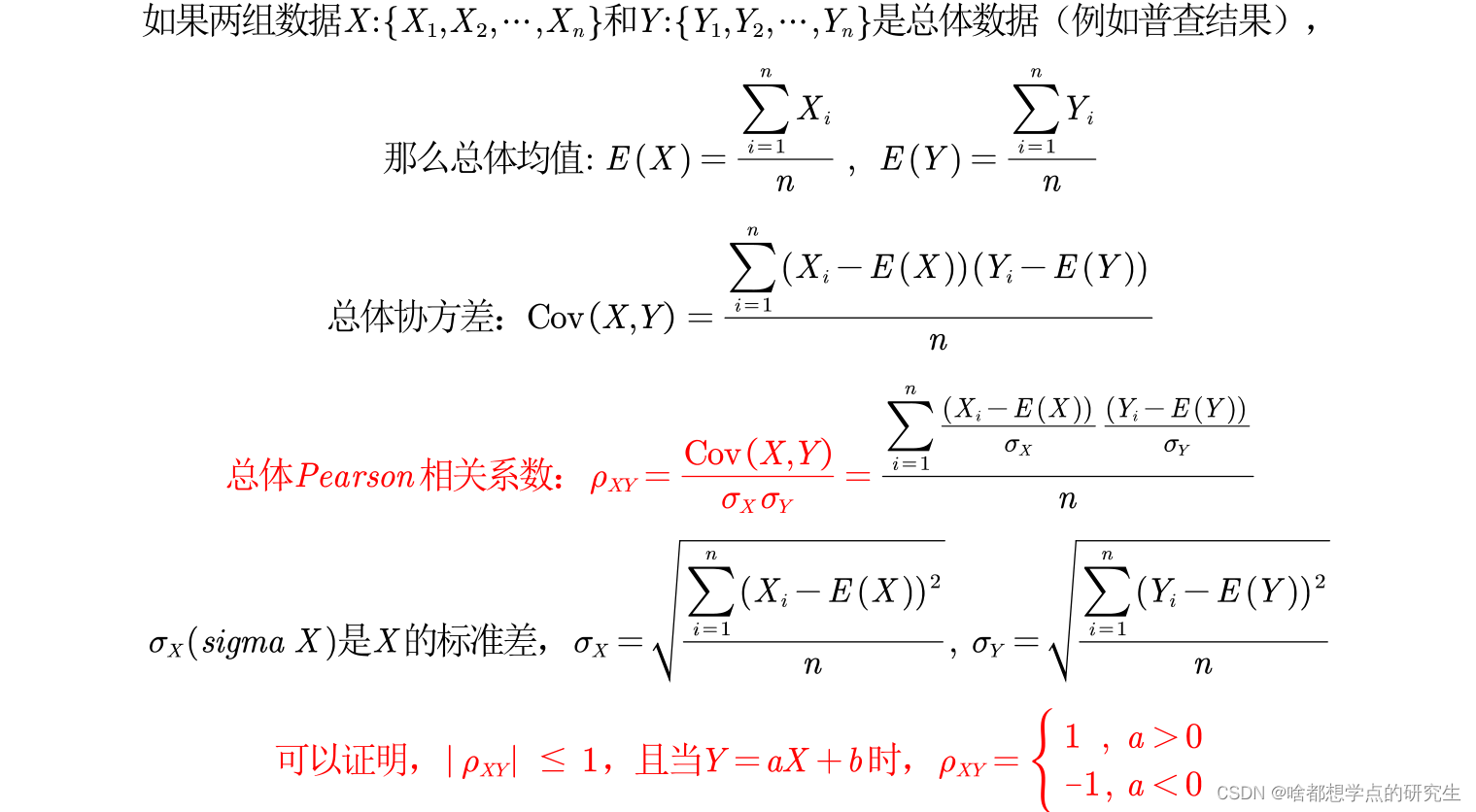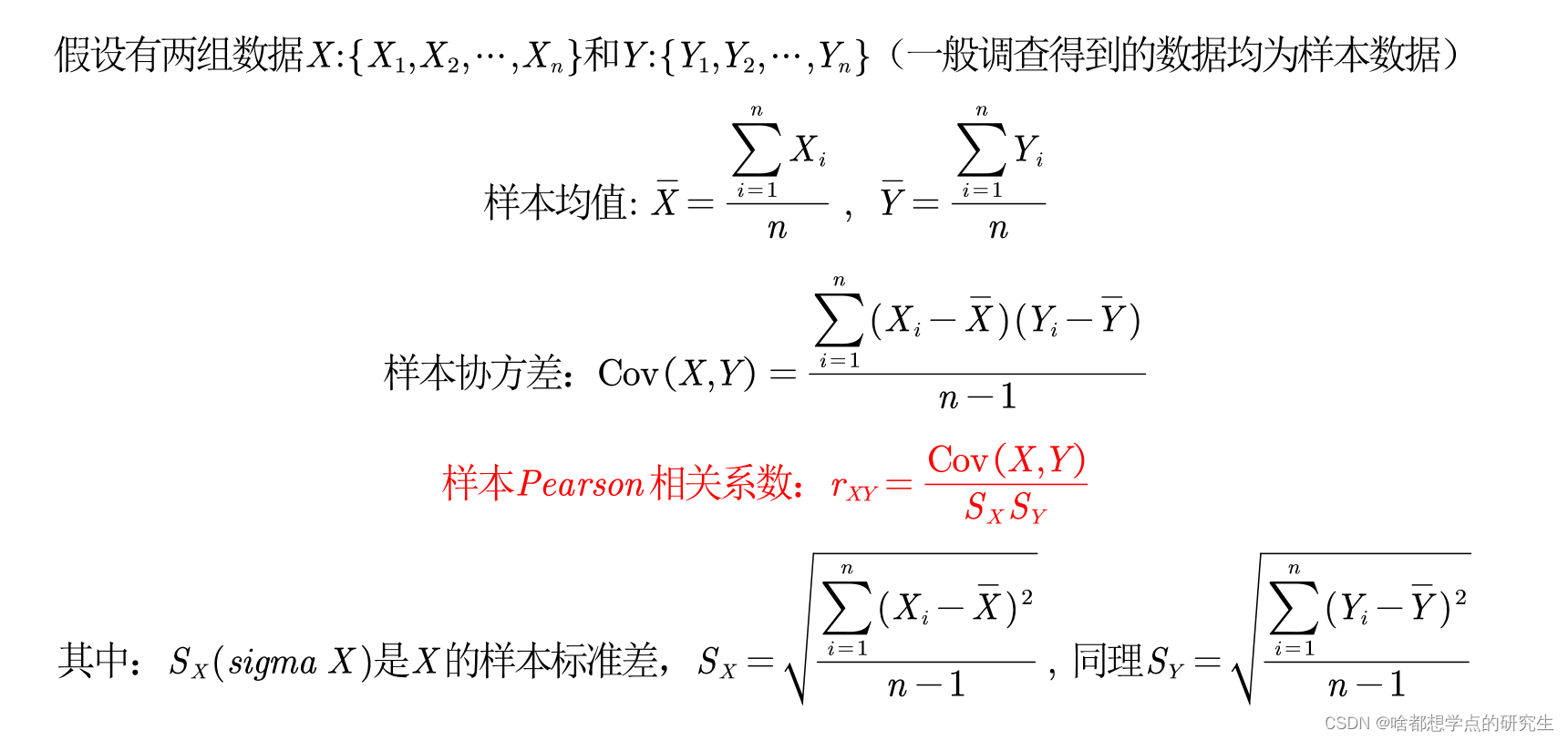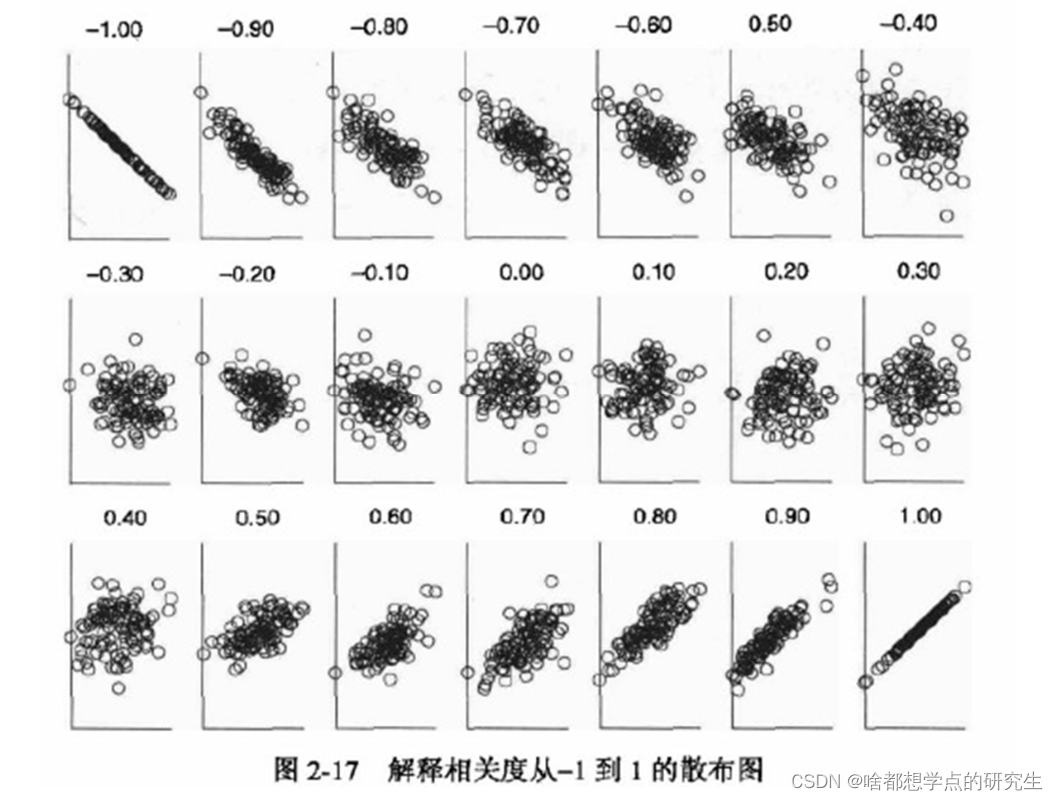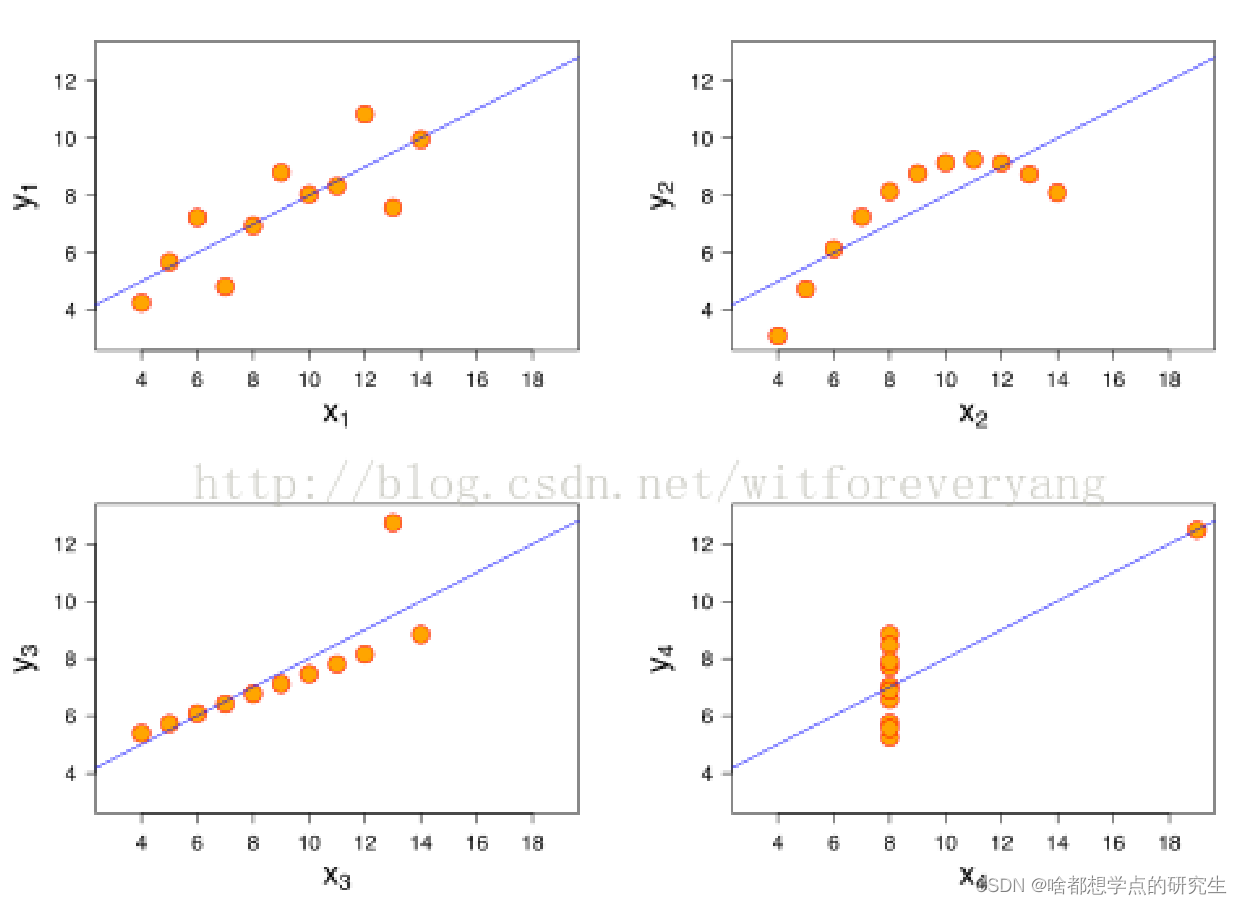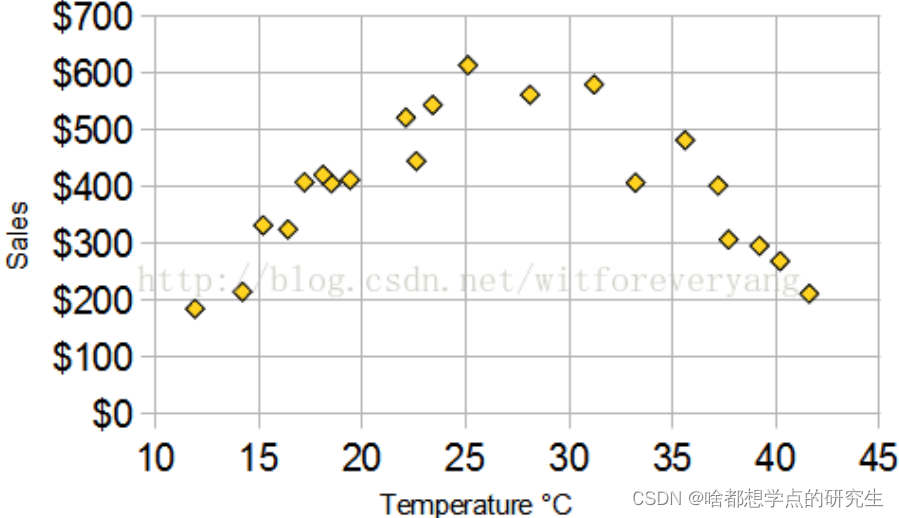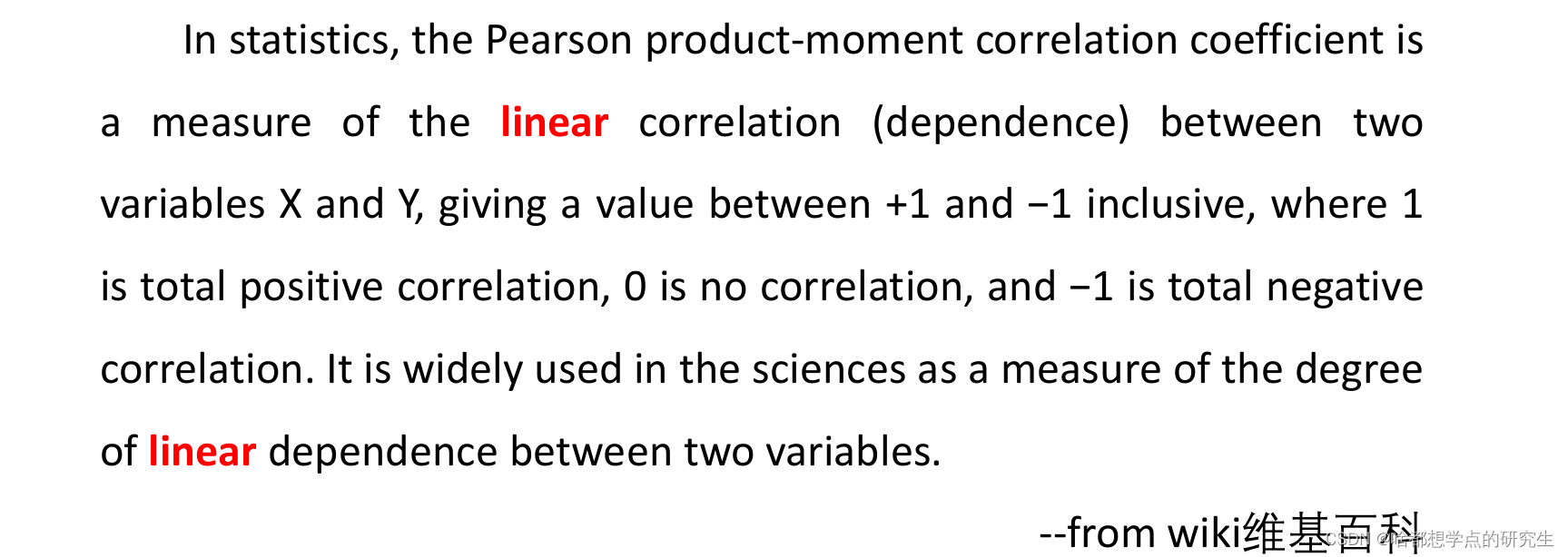（1）非线性相关也会导致线性相关系数很大，例如X2，Y2。
（2）离群点对相关系数的影响很大，例如X3,Y3，去掉离群点后，相关系数为0.98。
（3）如果两个变量的相关系数很大也不能说明两者相关，例如X4,Y4，可能是受到了异常值的影响。
（4）相关系数计算结果为0，只能说不是线性相关，但说不定会有更复杂的相关关系（非线性相关），例如冰淇淋与温度之间的关系。

（1）如果两个变量本身就是线性的关系，那么皮尔逊相关系数绝对值大的就是相关性强，小的就是相关性弱；
（2）在不确定两个变量是什么关系的情况下，即使算出皮尔逊相关系数，发现很大，也不能说明那两个变量线性相关，甚至不能说他们相关，我们一定要画出散点图来看才行。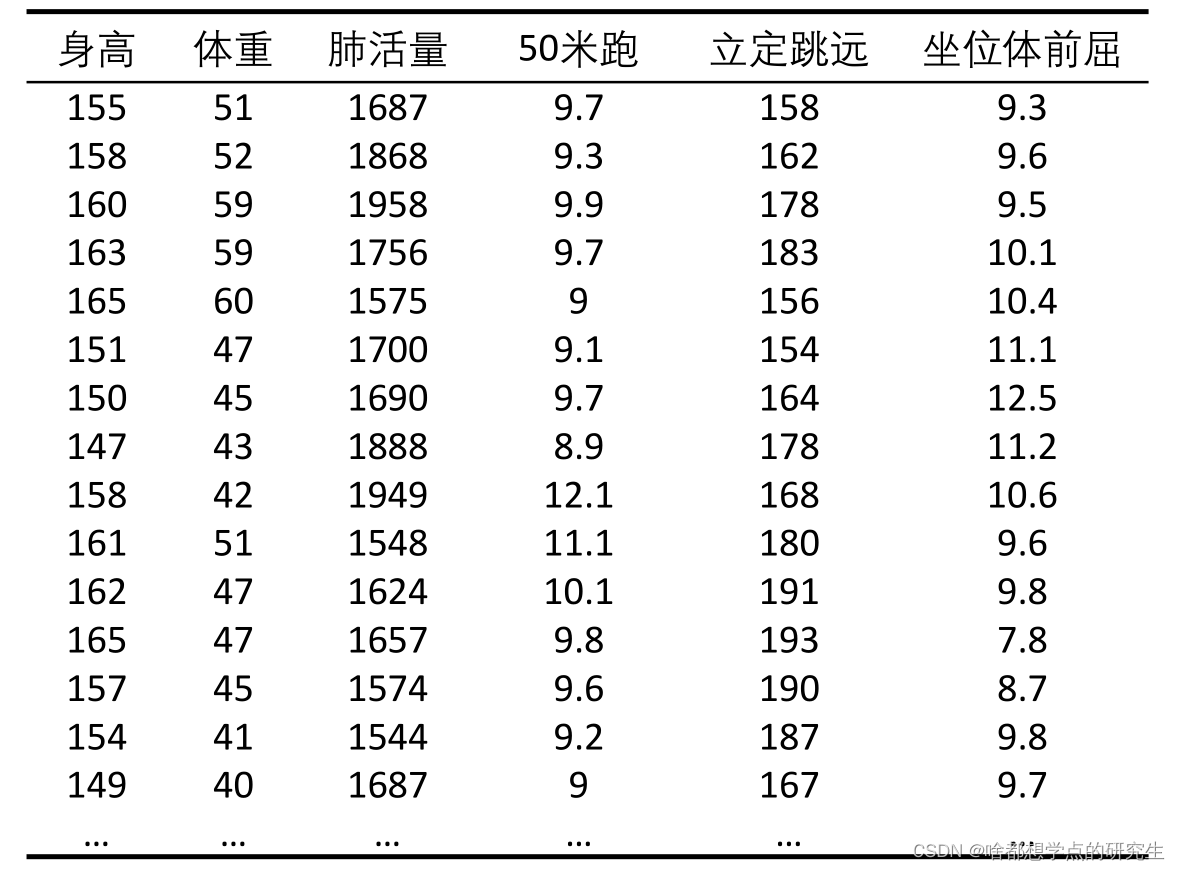Matlab中基本统计量的函数（一般用标粗的）：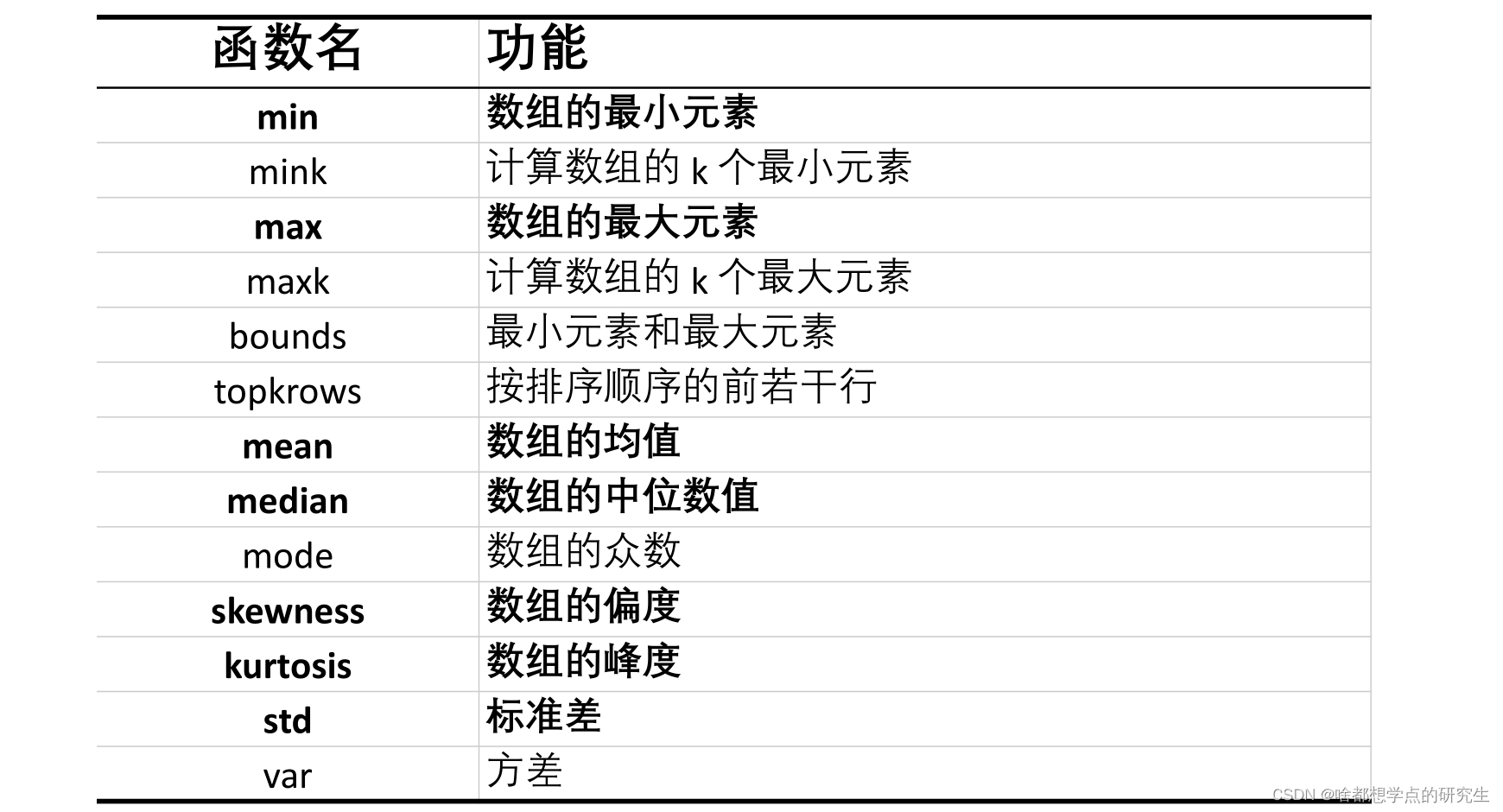clear;clc
load 'physical fitness test.mat' %文件名如果有空格隔开，那么需要加引号
%% 统计描述
MIN = min(Test); % 每一列的最小值
MAX = max(Test); % 每一列的最大值
MEAN = mean(Test); % 每一列的均值
MEDIAN = median(Test); %每一列的中位数
SKEWNESS = skewness(Test); %每一列的偏度
KURTOSIS = kurtosis(Test); %每一列的峰度
STD = std(Test); % 每一列的标准差
RESULT = [MIN;MAX;MEAN;MEDIAN;SKEWNESS;KURTOSIS;STD] %将这些统计量放到一个矩阵中表示

R = corrcoef(A)

R = corrcoef(A,B)

R = corrcoef(Test);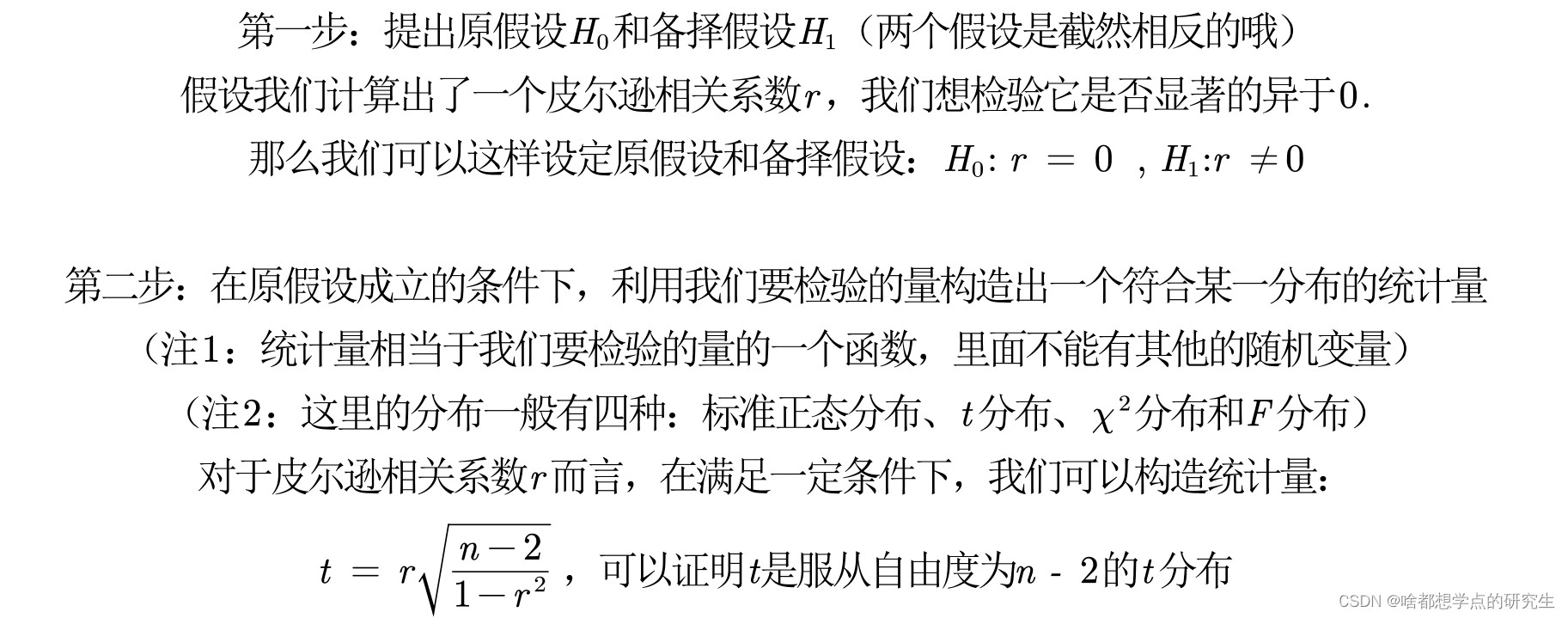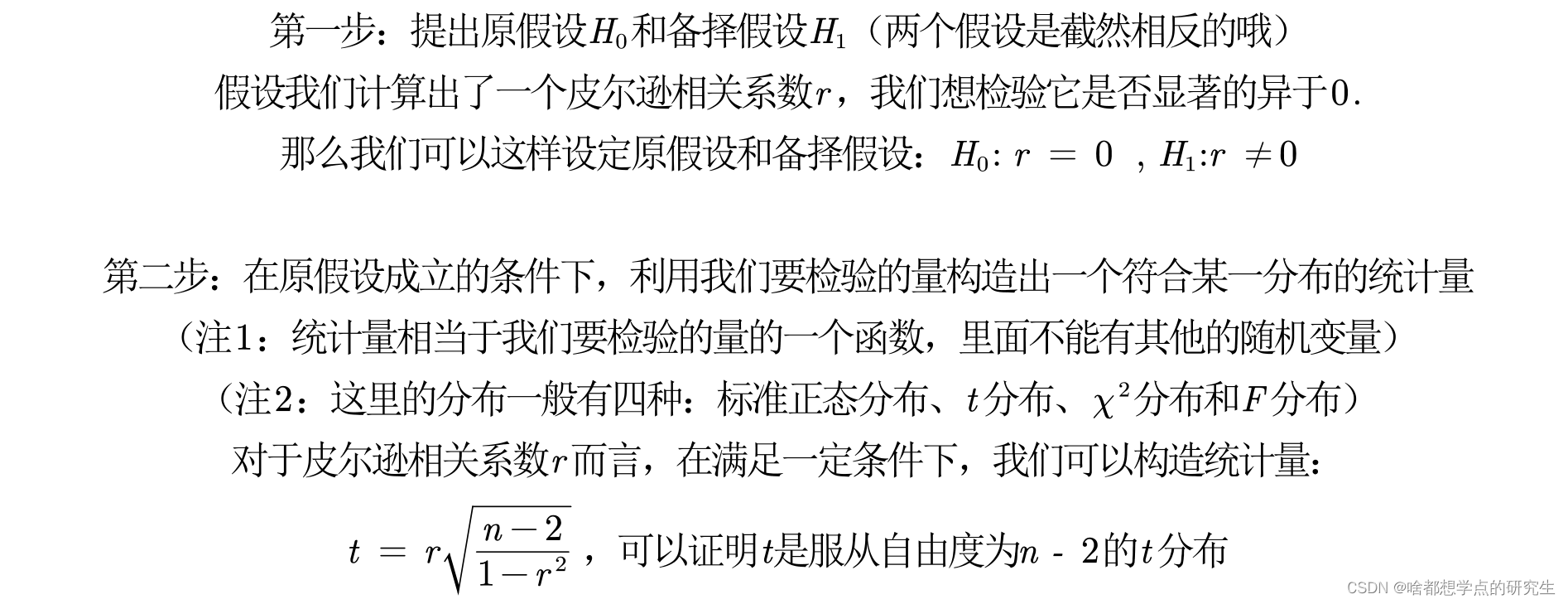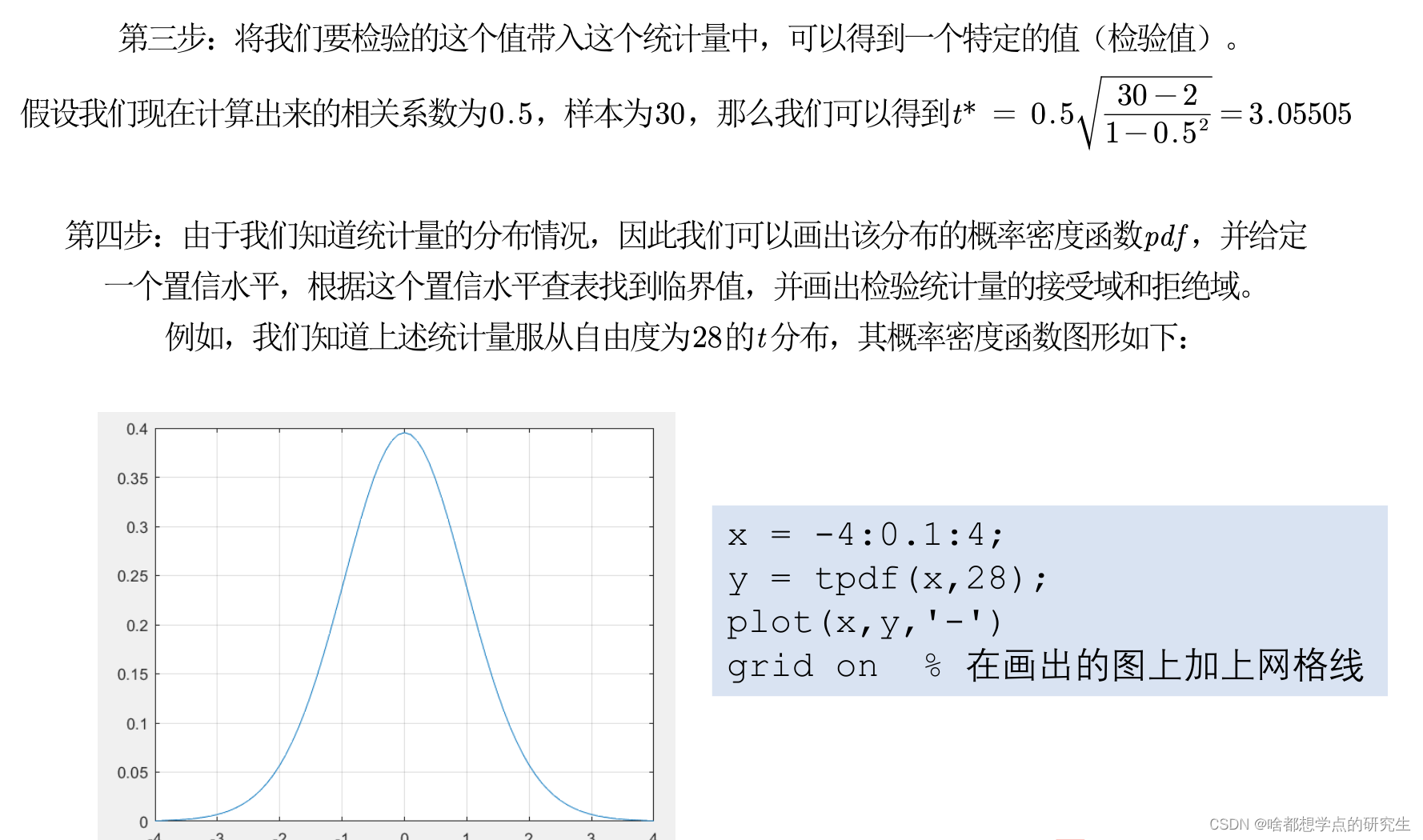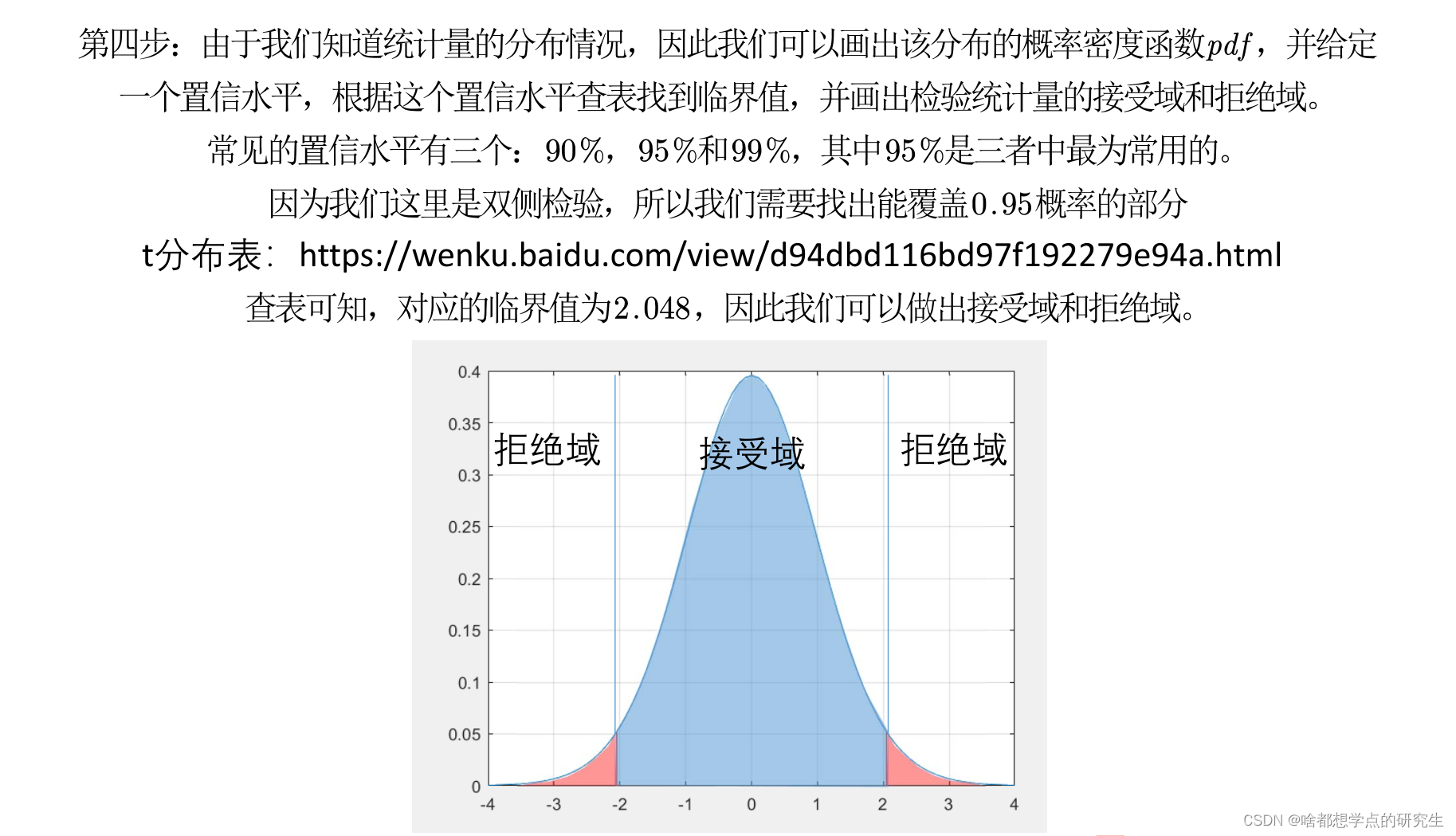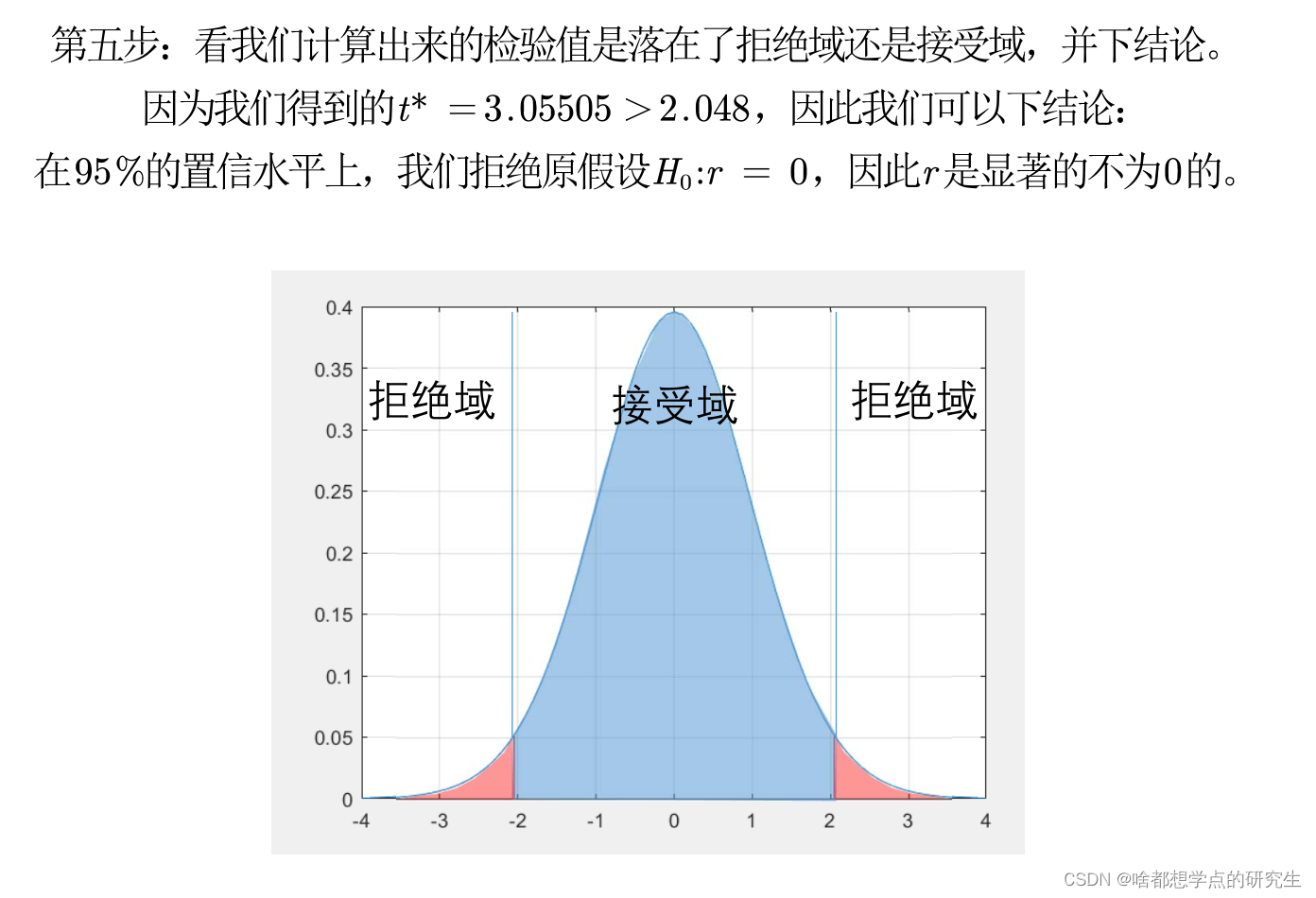P值判断法

%% 计算p值
x = -4:0.1:4;
y = tpdf(x,28);
figure(2)
plot(x,y,'-')
grid on
hold on
% 画线段的方法
plot([-3.055,-3.055],[0,tpdf(-3.055,28)],'r-')
plot([3.055,3.055],[0,tpdf(3.055,28)],'r-')
disp('该检验值对应的p值为：')
disp((1-tcdf(3.055,28))*2) %双侧检验的p值要乘以2

%% 计算各列之间的相关系数以及p值
[R,P] = corrcoef(Test)
% 在EXCEL表格中给数据右上角标上显著性符号吧
P < 0.01 % 标记3颗星的位置
(P < 0.05) .* (P > 0.01) % 标记2颗星的位置
(P < 0.1) .* (P > 0.05) % % 标记1颗星的位置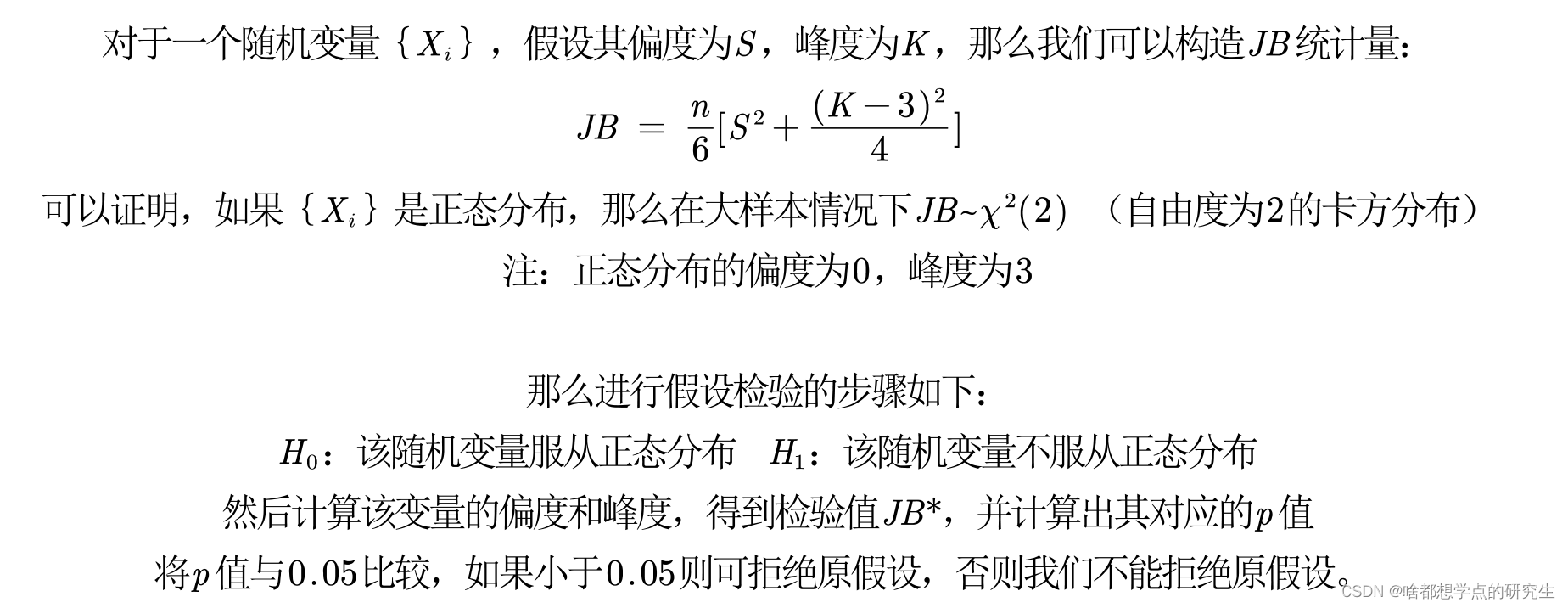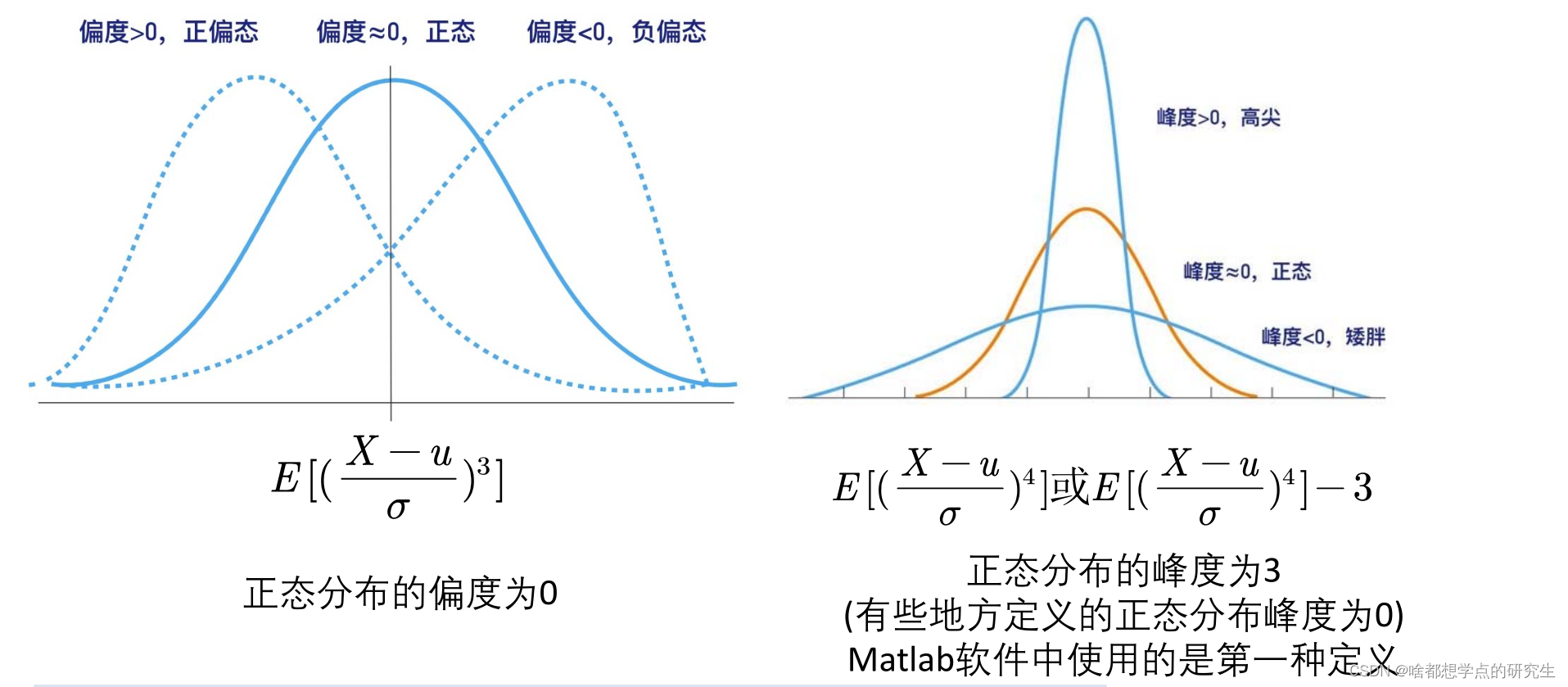% 正态分布的偏度和峰度
x = normrnd(2,3,100,1); % 生成100*1的随机向量，每个元素是均值为2，标准差为3的正态分布
skewness(x) %偏度
kurtosis(x) %峰度
qqplot(x)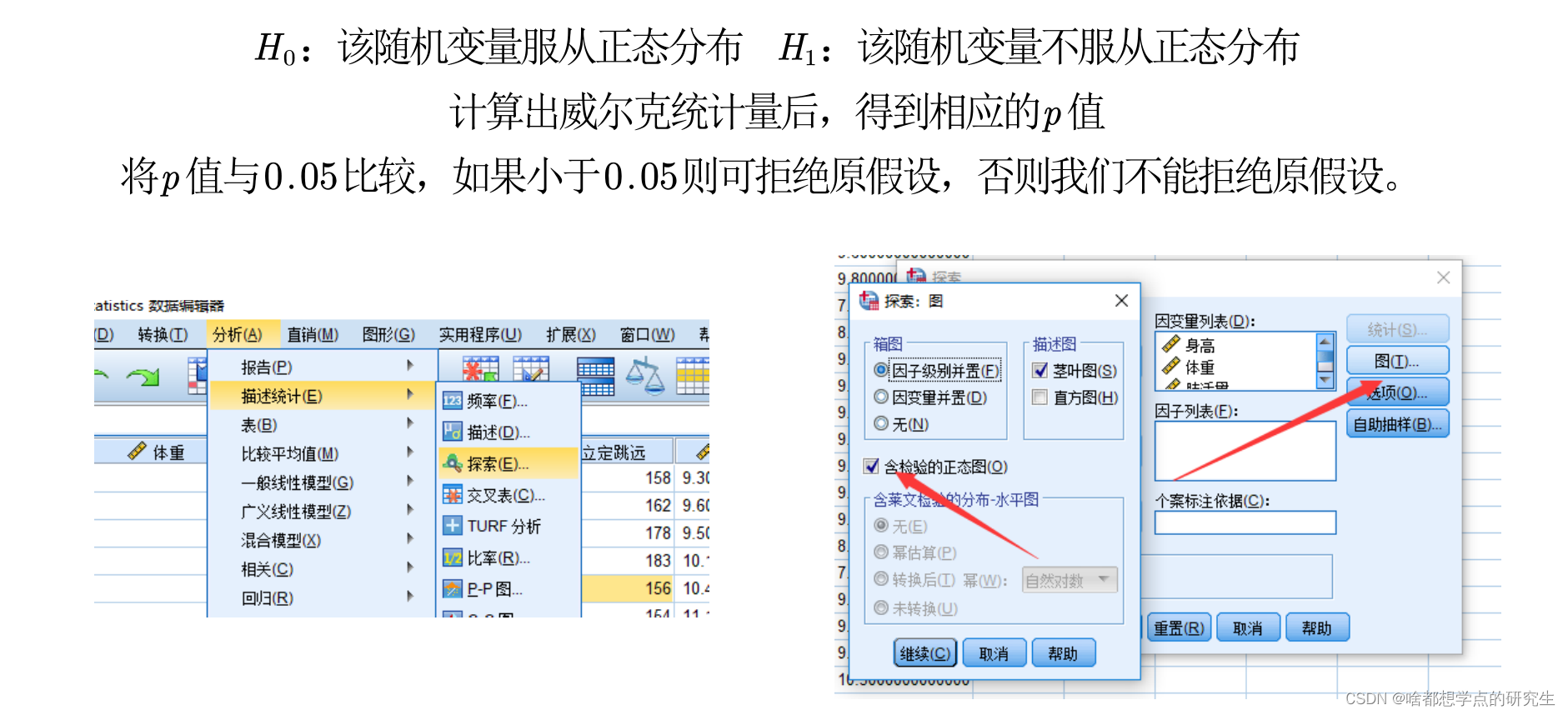QQ图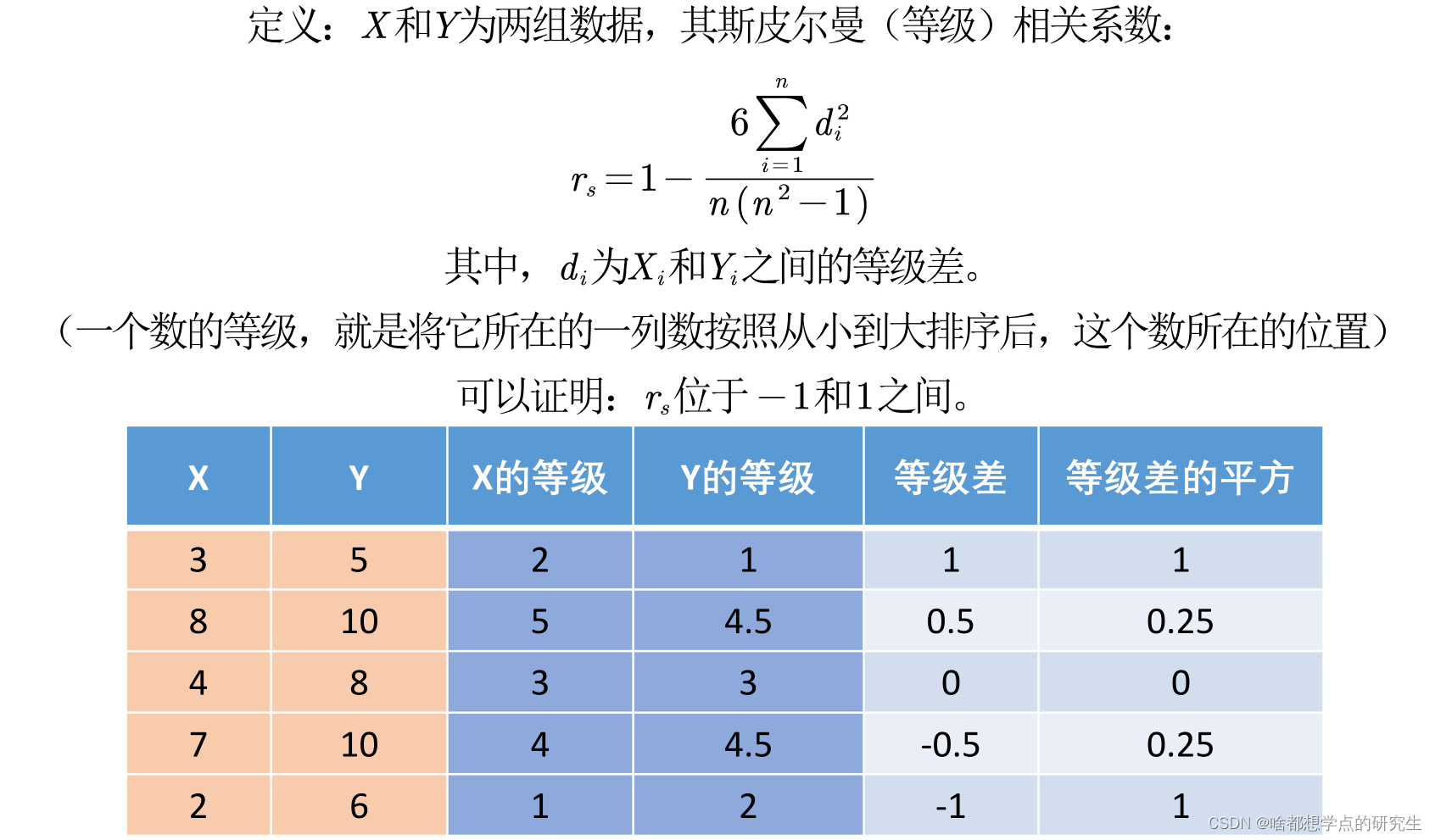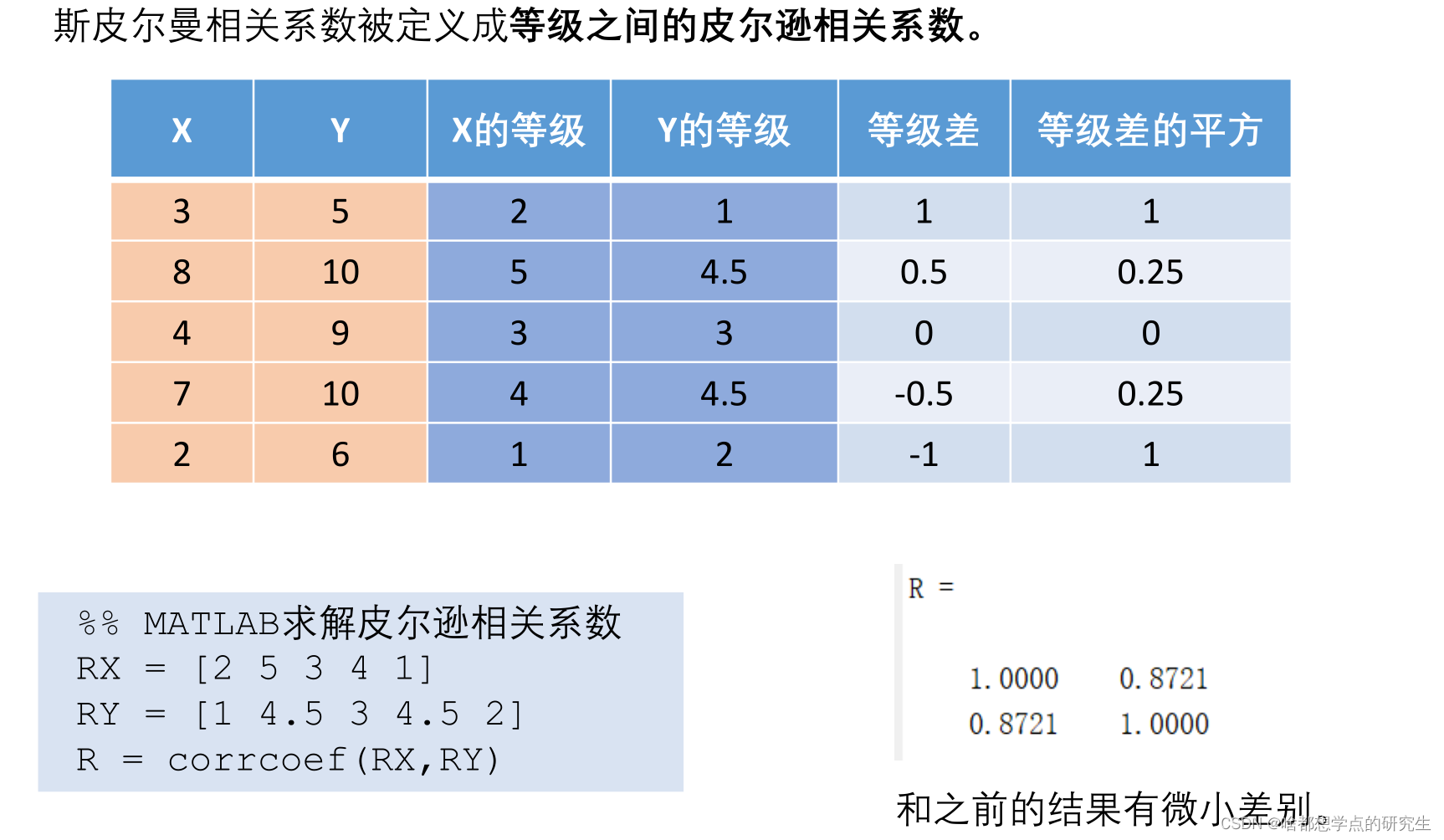1.连续数据，正态分布，线性关系，用pearson相关系数是最恰当，当然用spearman相关系数也可以， 就是效率没有pearson相关系数高。
2.上述任一条件不满足，就用spearman相关系数，不能用pearson相关系数。
3.两个定序数据之间也用spearman相关系数，不能用pearson相关系数。定序数据是指仅仅反映观测对象等级、顺序关系的数据，是由定序尺度计量形成的，表现为类别，可以进行排序，属于品质数据。

### “数学建模&mdash;&mdash;相关系数”的评论:

##### 关于作者##### overfit同步小助手